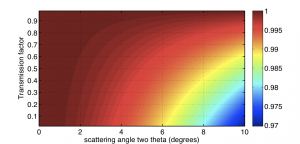# Does it matter? part 1: sample direction-dependent absorption

This series is part of a set to determine which corrections matter when. We all heard -or read- about corrections Small-angle Scatterers do not need to do, because they are supposedly negligible. Let’s look at some of them and determine if this is really true or not.

The first looks at the sample direction-dependent absorption. This is the effect of scattered radiation travelling a slightly different distance through the sample (and therefore experiencing different levels of absorption) depending on the direction of scattering. Take, for example, the simplest case of a sample like a plate or sheet (c.f. Figure 1).

From this figure, it can easily be seen that the radiation scattering to an angle has to travel longer through the sample than radiation passing straight through. Therefore, the scattered radiation suffers from more absorption. But how much more?

In this document: plate_transmission, with the help of Samuel Tardif, we calculated the exact angle-dependent absorption of a plate-like sample. The short answer, for those who do not want to read the document:Figure 2: Correction factor for different levels of absorption and different scattering angles

The sample absorption indeed accounts for less than a percent of deviation to a scattering angle of 10 degrees (degrees, as this effect is independent of the wavelength), if the sample absorption is lower than 75 % (which is already much more absorption than the max. 30% rule-of-thumb you were told by your senior scatterers). The full diagram is shown in Figure 2 (click to enlarge).

So, on the grand scale of things, this correction is indeed negligible for most samples (watch out for the highly absorbing ones though!). However, given its simplicity, it should not be much trouble to implement for sheet-like samples at least.

The correction is very straightforward to implement (see document) as you only need to know the transmission factor (which you knew anyway, because you measured it for the background subtraction, right?). This only applies to sheet-like or plate-like samples, the calculation for a capillary is a little bit more complicated.

The capillaries also suffer from this, but this plate-like absorption is the maximum correction to apply for capillary geometry (in the direction parallel to the capillary axis). So also for capillaries, implementation is unnecessary unless you are hunting for the final fractions of a percent accuracy.

Next time we discuss another one: the polarization correction!

#### 5 Trackbacks / Pingbacks

This site uses Akismet to reduce spam. Learn how your comment data is processed.